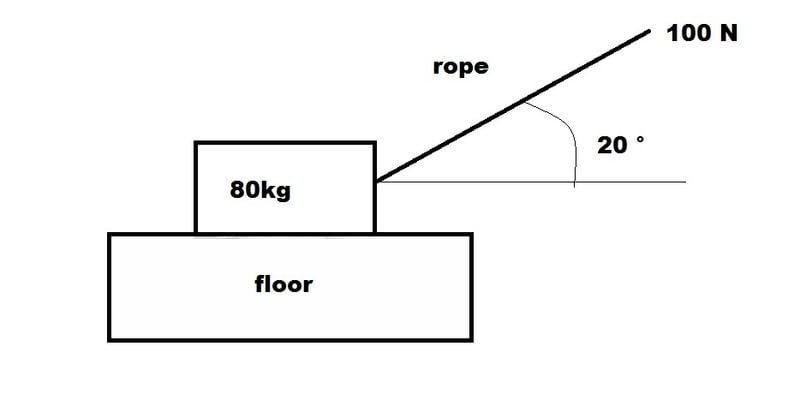# Help In Mechanics (DumB)

• shinnsohai
In summary, the box cannot be equilibrium if the floor is smooth because the floor creates a frictional force that is greater than the force that is needed to move the box.

## Homework StatementA box of mass 80kg is pulled along a horizontal floor by a rope.
The rope is pulled with a force of 100N and the rope is inclined at (20 °) to the horizontal

1. Explain Briefly why the box cannot be equilibrium if the floor is smooth.
(In Fact the floor is not smooth and the box is in equilibrium )

2. Calculate The Frictional Force Between The box and the floor and also the normal reaction of the floor on the box

The Maximum value of the frictional force between the box and the floor is 120N and the box is now pulled along the floor with the rope always inclined 20° to the horizontal

3. Calculate the force with which the rope must be pulled for the box to move at a constant speed.

4. Calculate the acceleration of the box if the rope is pulled with a force of 140N

F = ma
F= μR

## The Attempt at a Solution

1.Once after the force being applied of angle 20, the Normal force will also being bend 20 degree so that it is not in a equilibrium state (Correct Me If I am Wrong)

2.

x-axis

100 cos 20 - F = 0 ----1
F= -93.9693

y-axis
R+ 100 sin 20 - 40 =0
R = -14.2020

tan F/R = μ (Coefficient of Friction)

tan ( -93.9693 / -14.2020 )
μ = 0.115979928

3.Min Force to be applied

(80kg x 9.81 ) sin 20 = 268.4174
F = 268.4174N > 120 N ( already overcome the frictional force between the floor and the box)

4. Acceleration of the box ,140N applied

1)I'm not sure about your language here. What does 'Normal force will also being bend 20 degree' mean?

2)The equation for the x-axis is right. But I think the equation for the y-axis is wrong. For some reason, it looks like you've written the weight as 40N, but the mass is 80kg, so this can't be right.

The question doesn't ask for the coefficient of friction, and you don't need to use it in any later calculations, so there's no need to calculate it.

3)I don't understand what you tried to do here, and I don't think the answer is correct. You've been given the value of the friction force, so you should use this to calculate the value of the pulling force (keeping in mind that the object is moving at constant speed, so the total force should be zero).

4) You haven't started this one yet. You're given the pulling force, and you had already been given the friction force, so you can calculate the acceleration.